Intervals

Intervals are subsets of a universe, that are defined by upper and lower boundaries. Typically we think about real numbers, but any totally ordered universe allows the definition of intervals.

Intervals are defined by lower and upper boundaries, which can be a limiting number or unlimited, typically written as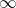for the upper bound andfor the lower bound. The boundaries can be included or excluded. So the following combinations exist for a universe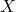: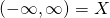unlimited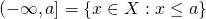half open, lower unlimited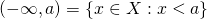open, lower unlimited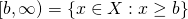half open, upper unlimited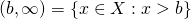open, upper unlimited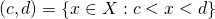openhalf open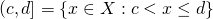half open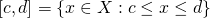closedit is sometimes useful to consider the empty set as an interval as well

The words „open“ and „closed“ refer to our usual topology of real numbers, but they do not necessarily retain their topological meaning when we extend the concept to our typical data types.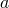,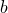,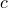andin the notation above do not have to be members of, as long as the comparison is defined between them and all members of. So we could for example meaningfully define for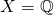the interval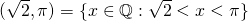.

As soon as we do not imply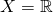we always have to make this clear… And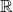is kind of hard to really work with in software on computers with physically limited memory and CPU power.

Intervals have some relevance in software systems.

We sometimes have a business logic that actually relies on them and instead programming somehow around it, it is clearer and cleaner to actually work with intervals. For example, we can have a public transport scheduling system and we deal with certain time intervals in which different schedules apply than during the rest of the day. Or we have a system that records downtimes of servers and services and these are quite naturally expressed as intervals of some date-time datatype. It is usually healthy to consider all the cases mentioned above rather than ignoring the question if the boundary with probability zero of actually happening or having ugly interval limits like 22:59:59.999.

The other case is interval arithmetic. This means, we do floating point calculations by taking into account that we have an inaccuracy. So instead of numbers we have intervals. When we add two intervals, we get. In the same way we can multiply and subtract and even divide, as long as we can stay clear of zero in the denominator. Or more generally we can define.
It does of course require some mathematical thinking to understand, if the result is an interval again or at least something we can deal with reasonably. Actually we are usually happy with replacing the result by an interval that is possibly a superset of the real result, ideally the minimal superset that can be expressed with our boundary type.

At this point we will probably discover a desire to expand the concept of intervals in a meaningful way to complex numbers. We can do this by working with open disks likeor closed disks like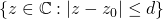. Or with rectangles based on two intervalsand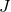like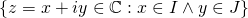.

These two areas are quite interesting and sometimes useful. Libraries have been written for both of them.

Often we discover, that intervals alone are not quite enough. We would like to do set operations with intervals, that is

union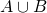intersection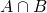set difference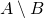While the intersection works just fine, as long as we include the empty setas an interval, unions and differences lead us to non-intervals. It turns out that interval-unions, sets that can be expressed as a union of a finite number of intervals, turn out to be a useful generalization, that is actually what we want to work with rather than with intervals. In this case we can drop the empty set as interval and just express it as the union of zero intervals.

There are some questions coming up, that are interesting to deal with:

normalization
Can we normalize interval-unions to some canonical form that allows safe and relyable comparison for equality?
is our universeactually discrete, so we can express all unlimited boundaries with closed boundaries?
interval lengths
Do we have a meaningful and useful way to measure the length of an interval or the total length of an interval-union, as long as they are limited? Or even for unlimited intervals?
collection interfaces
Do we want to implement a Set-interface in languages that have sets and an understanding of sets that would fit for intervals
implementation
How can we implement this ourselves?
implementation
Can we find useful implementations?

Having written a java library to support interval-unions on arbitrary Comparable types once in a project and having heard a speech about an interval library in Scala that ended up in using interval-unions in a pretty equivalent way, it might be interesting to write in the future about how to do this or what can be found in different languages to support us. For interval arithmetic some work has been done to create extensions or libraries for C and Fortran, that support this, while I was a student. So this is pretty old stuff and interesting mostly for the concepts, even if we are not going to move to Fortran because of this.

If there is interest I will write more about actual implementations and issues to address when using or writing them.